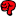## tmax in case of ties: R vs. R vs. SAS [Nonparametrics]

Dear all!

Ok, this thread could also go into the category "R for BE/BA". But this here is Helmut's favorite.

Despite the fact that non-parametrics will no longer be accepted by the EMAthe world is bigger than Europe and many guidelines still require a non-parametric analysis of tmax (some of them obviously copied from the old EMEA guidance).

It is well known1), that the analysis in case of the classical 2x2 cross-over is done by analysing the period differences (P1-P2) via a Wilcoxon rank sum test with the sequence as grouping factor.

Since in case of tmax there are usually some/many equal values (ties in the non-parametric methods speak) the used software should be able to handle such.
In exploring this within R using the tmax values from the single dose data of Bear I came up with:
# Bear single dose data data <- c("  subject sequence      P2     P1        1       TR      2.0    2.0        2       RT      1.5    3.0        3       TR      2.0    2.0        4       RT      2.0    2.0        5       TR      3.0    2.0        6       RT      3.0    2.0        7       TR      1.5    2.0        8       RT      3.0    2.0        9       TR      2.0    3.0       10       RT      2.0    1.5       11       TR      1.5    2.0       12       RT      2.0    3.0       13       TR      1.5    3.0       14       RT      3.0    3.0") tc <- textConnection(data) PKt  <- read.table(tc, header=TRUE, strip.white=TRUE, as.is=TRUE) close(tc) PKt$pdiff <- PKt$P1 - PKt$P2 # to run it again we must remove coin if ("package:coin" %in% search()) detach("package:coin",unload=TRUE) library(exactRankTests) t1 <- wilcox.exact(pdiff ~ sequence, data=PKt, conf.int=TRUE, conf.level=0.9) print(t1) This tolds me:  Package 'exactRankTests' is no longer under development. Please consider using package 'coin' instead. Exact Wilcoxon rank sum test data: pdiff by sequence W = 18, p-value = 0.4149 alternative hypothesis: true mu is not equal to 0 90 percent confidence interval: -1.5 0.5 sample estimates: difference in location -0.25 BTW: Because of lexical ordering RT vs. TR is tested and this estimates R-T. Thus we have to change the sign and exchange upper and lower bound to get them for T-R in which we are interested. Since I'm an obedient man (especially if my wife speaks to me) I followed the polite suggestion got after loading package 'exactRankTests' and tried: library(coin) PKt$sequence <- as.factor(PKt\$sequence) #very important t2 <- wilcox_test(pdiff ~ sequence, data=PKt, conf.int=TRUE, conf.level=0.9,                   distribution="exact") print(t2) 
and this told me:
    Exact Wilcoxon Mann-Whitney Rank Sum Test data:  pdiff by sequence (RT, TR) Z = -0.8465, p-value = 0.4149 alternative hypothesis: true mu is not equal to 0 90 percent confidence interval:  -1.5  0.5 sample estimates: difference in location                   -0.5

Upps! Different point estimates.
In other examples (no room to show here) with tied data I have also observed different CIs!

Any body out there who knows whats going on here?
I have searched the R support lists and found that observed by others. But the answers where at least not sufficient if not meaningless.
I have found a stat. course website which states "There is an R function wilcox.exact ... It does not do confidence intervals or point estimates correctly in the presence of ties." but without an explanation.

The new option HL (=Hodges Lehmann estimator) in my "Power to knoff" SAS® 9.2 Proc NPAR1way (code upon request) gives me between other stuff:
             The NPAR1WAY Procedure ...         Average scores were used for ties.            Wilcoxon Two-Sample Test              Statistic (S)               59.0000              Exact Test              One-Sided Pr >=  S           0.2075              Two-Sided Pr >= |S - Mean|   0.4149 ...              Hodges-Lehmann Estimation              Location Shift    0.5000                                                 Interval        Asymptotic Type                 90% Confidence Limits      Midpoint    Standard Error Asymptotic (Moses)     -0.5000      1.5000        0.500            0.6080 Exact                  -0.5000      1.5000        0.500
but this estimates T-R because TR is tested versus RT here.

1)Hauschke et.al.
"A distribution free procedure for the statistical analysis of bioequivalence studies"
Int. J. Clin. Pharmacol. Vol. 28 (2) 1990, 72-78/Vol. 30, Suppl. 1, S37-43

Sorry for that lengthy post. But I intended to give the Rusers among us the possibility to verify my results with cut and paste.

Sorry2: I have totally forgotten that the estimate and the confidence interval calculated as given are for 2*(T-R). Thus the results must be divided by 2! But what I wanted to show remains.

Regards,

DetlewIng. Helmut Schütz